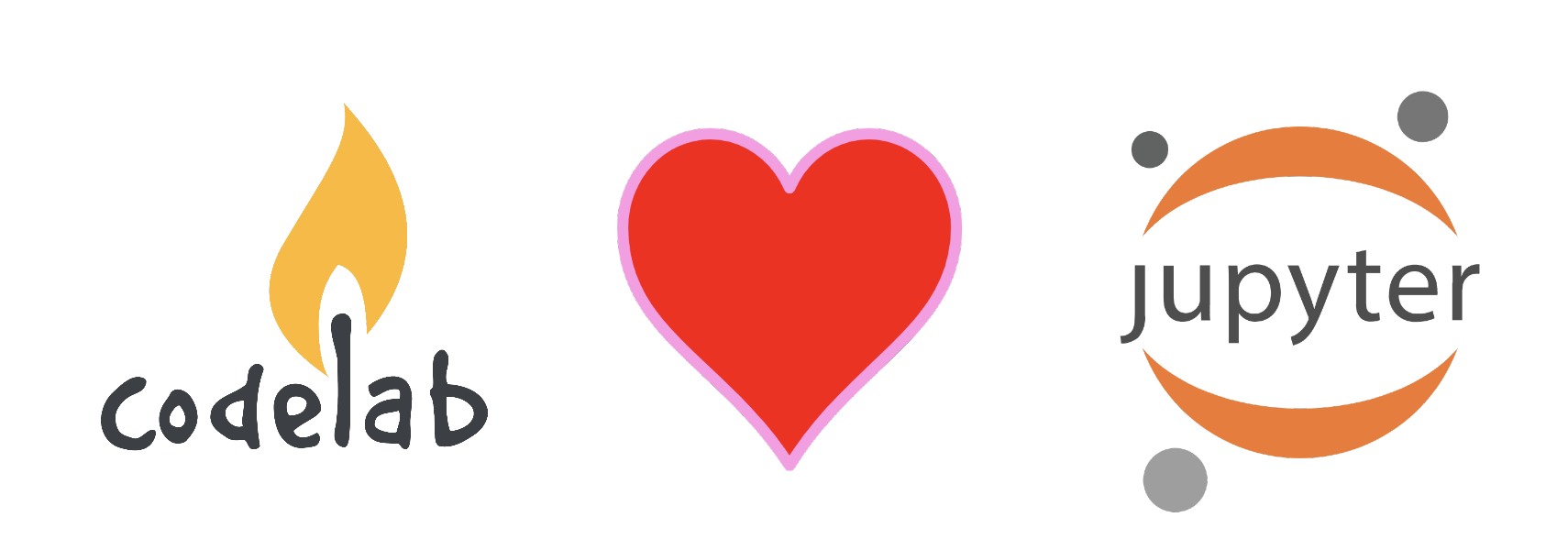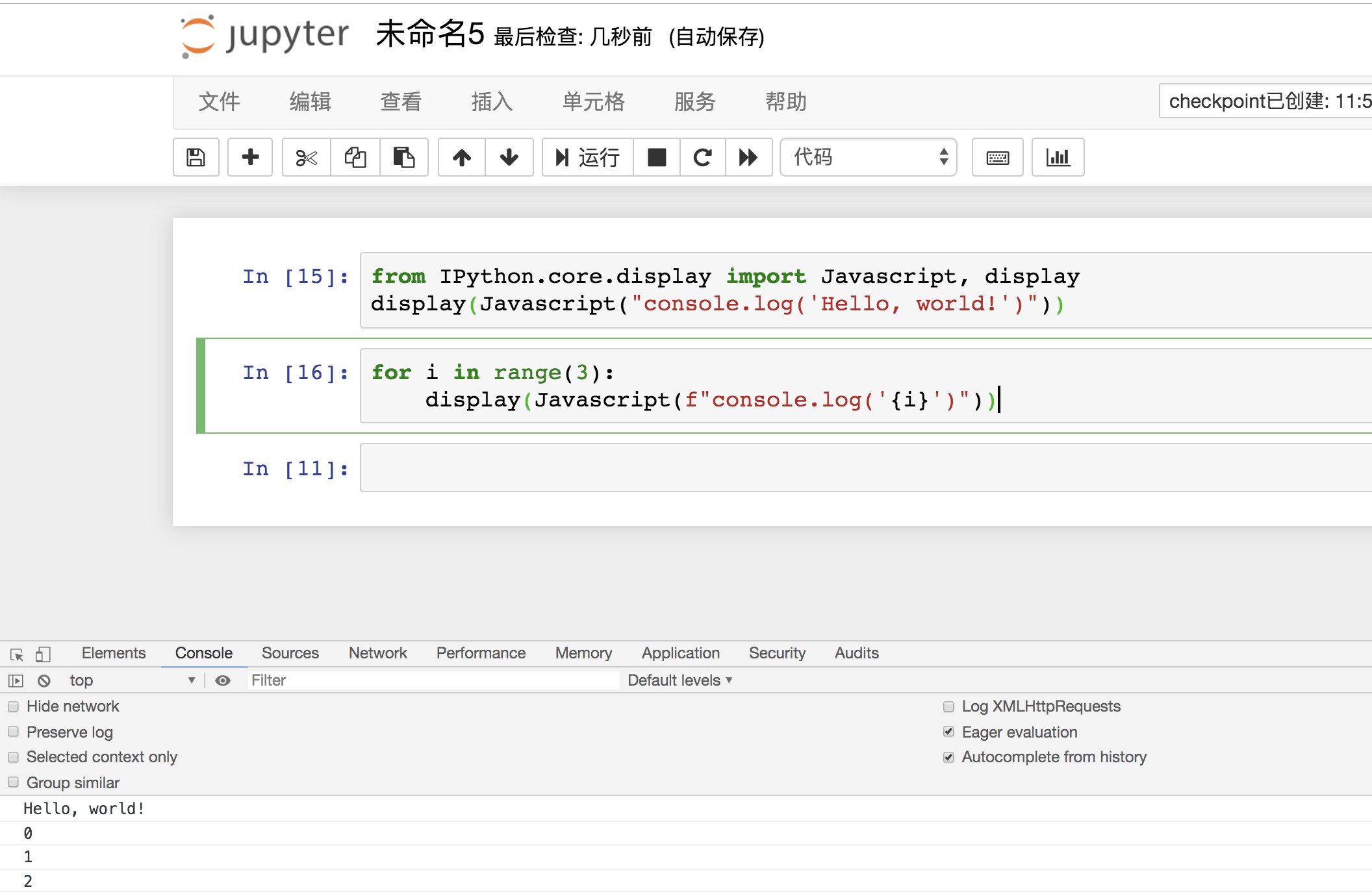CodeLab Adapter ❤️ Jupyter/Pythondemo

from codelab_adapter_client import SimpleNode
node = SimpleNode()

node.simple_publish("开启音乐")

node.simple_publish("下一个造型")

import time
for i in range(10):
time.sleep(0.2)
node.simple_publish("落下礼花")

# 完整演奏
import time
node.simple_publish("开启音乐")
time.sleep(0.2)
for i in range(30):
time.sleep(0.2)
node.simple_publish("下一个造型")
time.sleep(0.2)
for i in range(5):
node.simple_publish("落下礼花")

更多的想象空间

Jupyter是目前最理想Python/数据分析/人工智能的编程教育环境。关于Jupyter在教育上的意义可以参考使用IPython Notebook来学习编程

CodeLab Adapter 1.0致力于将万物带入Scratch，实现education as life的目标。CodeLab Adapter 2.0则将万物带入到Python教育中。物联网、人工智能、开源硬件，你现在可以使用Python在Jupyter中与这些东西交互。

机器人教学

from codelab_adapter_client.topic import JUPYTER_TOPIC

def __init__(self):
super().__init__()
self.EXTENSION_ID = "eim/vector"
self.message = {"topic": JUPYTER_TOPIC, "payload": {"content": "robot.behavior.say_text('hello')"}}

def forward(self,distance,speed):
self.message["payload"]["content"] = f"robot.behavior.drive_straight(anki_vector.util.distance_mm({distance}), anki_vector.util.speed_mmps({speed}))"
self.publish(self.message)
def turn_left(self,degrees,speed):
self.message["payload"]["content"] = f"robot.behavior.turn_in_place(anki_vector.util.degrees({degrees}), speed=anki_vector.util.degrees({speed}))"
self.publish(self.message)

def say(self,content):
self.publish(self.message)

robot = MyRobotNode()
robot.forward(100,100)
robot.turn_left(90,180)
robot.say("hello")

from pypinyin import  lazy_pinyin
pinyin = "".join(lazy_pinyin('肚子 好饿 去吃 午饭啦'))
print(pinyin)
robot.say(pinyin)

线上学习平台

@曾老师近期在参与一个AI线上学习平台的构建，将基于Open edX + Jupyter

Open edX提供了绝佳的学习系统，也许是目前全球最强大的学习系统，它拥有绝佳的开放性和丰富的生态。而Jupyter则是目前最理想的Python//数据分析/机器学习/AI编程工具。@曾老师希望结合两者，为学习者提供舒适而高效的学习环境，初学者不必为环境的搭建焦头烂额，目前斯坦福大学和UC伯克利都在使用Open edXJupyter作为编程教育平台。

from IPython.core.display import Javascript, display
for i in range(3):
display(Javascript(f"console.log('{i}') //websocket send/recv message <-> CodeLab Adapter 2.0"))参考